# Maths - Topological Space

### Topological Space

 We can think of topology as a generalisation of geometry where we no longer have to use the concept of distance (metric space). Topology has other, more general concepts of nearness. Topology does not to be geometric though, it is applicable in many situations where concepts such as neighborhood, convergence and continuity can be used. For example sequences and recursively defined structures in computer science.So topology links together many aspects of mathematics such as set theory, logic and geometry.

We start with geometry because our human intuition can best understand concepts like neighborhood, convergence and continuity in geometric terms. So, for instance we can study 'rubber sheet geometry' or the properties of manifolds that are preserved by continuous maps.

To do this we use the concept of 'open space' and 'open set'. The notion of an open set provides a way to speak of distance in a topological space, without explicitly defining a metric on the space. Although one cannot obtain concrete values for the distance between two points in a topological space, one may still be able to speak of "nearness" in the space, thus allowing concepts such as continuity to translate into the theory of open sets.

## Topology on a Set

If we start out with a set, say {a,b,c}, we can define various topologies on that set:

Here is a non-precise definition of topology:

A topology on a set X is a collection τ (tau) of subsets of the powerset(X), called open sets satisfying the following properties:

• X and Ø are elements of τ.
• τ is closed under finite intersections.
• τ is closed under arbitary unions.

The elements of τ are called the open sets of the topology.

The pair (X,τ) is called the topological space.

### Examples of Topological Spaces

discrete and trivial are two extreems:

#### discrete space

The open sets are the whole power set.

The points are isolated from each other.#### trivial topology

The only open sets are the empty set Ø and the entire space.

The points are so connected they are treated like a single entity.The interesting topologies are between these extreems.

## Subdividing Space

 Sometimes, in mathematics, we deal with objects that are unbounded: we can keep increasing them indefinitely. For example, we could start with the quantity 1 then add one to that, then add one to that, and so on. If we take the limit to this we get infinity.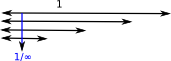Topology seems to be concerned more with the other direction, that is, if we start with a quantity (say 1) and then keep dividing it there is a infinitesimally small limit.

So subdividing an object is an important concept in topology.

### Subtopologies

We can generate sub-topologies τ' by taking a subset of the set X. The induced topology is:

τ' = (τX')

### Hausdorff Spaces

Topology has historically arisen from geometry, typically geometric situations use the 'Housdorff separation axiom'. Applications in computer science tend to be non-Hausdorff.

 Hausdorff - geometricThis defines the opposite of nearness (distinct points) non-Hausdorff - computer science (approximation)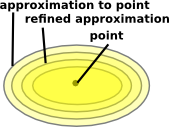This defines nearness.

A topological space is said to be a Hausdorff space if given any pair of distinct points p1, p2H, there exists neighborhoods U1 of p1 and U2 of p2 with U1U2 = Ø.

In other words: disjoint open sets separate points.

### Basis and Subbasis

A base (or basis) B for a topological space X with topology τ is a collection of open sets in τ such that every open set in τ can be written as a union of elements of B. We say that the base generates the topology τ.

• Every open set is a union of basis elements.
• Every open set is a union of finite intersections of subbasis elements.

### Fibre Bundles and sheafs

The concept of a 'fibre bundle' allows us to define some structure to subsets of a space and the 'sheaf' concept adds more structure.

 Topology allows us to look at mappings of points (shown at the top of this diagram) and the mapping between neighborhoods (open sets). The mapping between the logic of open sets is in the reverse direction (contravarient) and defines the continuity.If I is a set: I=set{A,B,C,D}
then a bundle is denoted Bn(I) or setI
Wheredenotes a comma category.

Topology Category Objects Morphisms Logic
Fibre Bundle BN(I)
equivalent to comma category:
setI
pairs:
(A,f)
where
f:A->I
(A,f) -> (B,g)
preserves meets and joinsall subsets of I
sheaf TOP(I)   preserves meets and joins and subsets (implication)
that is Heyting algebra.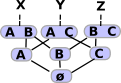open subsets of I

### Homeomorphisms

 A homeomorphism preserves 'nearness' but allows 'rubber geometry' deformations. Note: homeomorphism is a different concept from homomorphism.### Projections

 We can project helix onto circle and still keep continuity.## Algebraic Topology

In two dimensions it is relatively easy to determine if two spaces are topologically equivalent (homeomorphic). We can check if they:

• are connected in the same way.
• have the same number of holes.

However, when we scale up to higher dimensions this does not work and it can become impossible to determine homeomorphism. There are methods which will, at least, allow us to prove more formally when topological objects are not homeomorphic.

These methods use 'invariants': properties of topological objects which do not change when going through a homeomorphism. Here we look at two types of invariants which arise from homotopy and homology.

### Homotopy

It would take up too much space to properly explain the concept here so see this page for more details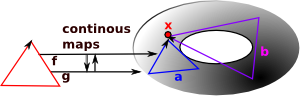In homotopy we use equivalence classes between a circle and loops (which don't collapse) on the topological object that we are investigating. We can get further invariants by extending the circle to the surface of higher order n-spheres.

We can then get algebraic structures (mostly groups) by investigating what happens when these loops are composed, the loops are generators of the group. In homotopy the order of these compositions can be significant, that is the groups are not necessarily abelian.

### Homology

It would take up too much space to properly explain the concept here so see this page for more detailsIn homology we dont just use n-spheres but every closed oriented n-dimensional sub-manifold.

It also uses a different definition of equivalence classes where composition of loops commutes. This results in abelian groups.

So we dont need to fix the basepoint.

### Topology on a Set

A topology on a set X is a collection Τ of subsets of X, called open sets satisfying the following properties:

• X and Ø are elements of Τ.
• Τ is closed under finite intersections.
• Τ is closed under arbitrary unions.

The requirements for the existence of of meets and joins correspond to the requirements for the existence of unions and intersections of open sets. Therefore these lattice structures can represent topologies.

Lattice Open Set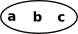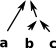invalid - every intersection should be an open set: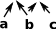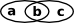Venn Diagram Topological Space (or not) Lattice (frame)This is not a topological space because 'a' and 'b' are subsets but not the union of 'a' and 'b'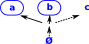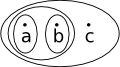This is now a topological space because we have added the union of 'a' and 'b'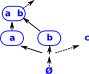This is not a topological space because 'ab' and 'bc' are subsets but not their intersection.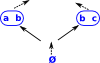This is now a topological space because we have added 'b'Note: Assume Ø is included in the above examples.

### Open Set Criterion for Continuity

A map ƒ: M1 → M2 is continuous if and only if the inverse image of every open set is open.

## Link between Topology and Logic

 One way to visualise the link between topology and logic is to start with a Venn diagram. We can then map points in the Venn diagram to either true or false depending on whether they are in a given set.This is a nice way to link geometry, logic and topology.

See page here.

See page here.

## Examples

### Non-Geometric Examples

#### Sequences

Here the concept of 'nearness' comes from sequences that begin in the same way.